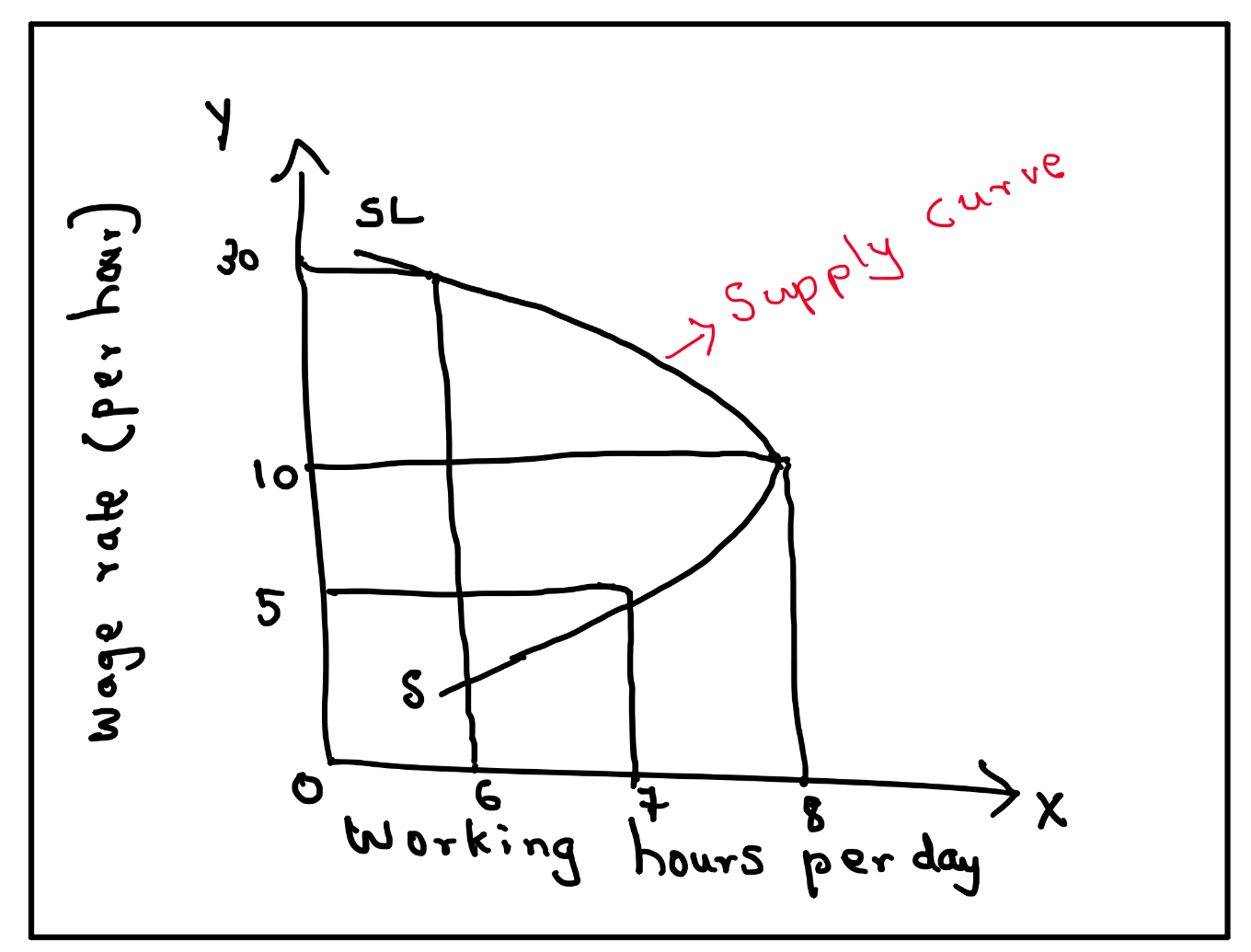9TH MAHARASHTRA: MATHS I 10TH TAMIL NADU: MATHS, SCIENCE, SSLC 10TH NEW TEXT BOOK PDF DOWNLOAD CBSE 10TH: MATHS 2019 BOARD PAPER SOLUTION: 2019 BOARD PAPER SOLUTION

Supply curve of labour bends backwards.

Reasons: Yes, I do agree with the statement.

(i) When wage rate rises supply of labour also rises up to some extent but at higher wage rate labour prefer rest to the work therefore supply curve of labour is backward bending as shown in the following diagram.ii. OX axis represent working hours per day and OY axis represents wages rate ‘SSL’ is supply curve of labour, which is backward bending it shows that when wage rate is Rs. 5/- per hour labour works for 7 hours per day. When wage rate rises to Rs. 10/- per day labour works for 8 hours in a day and supply of labour is extended.

iii. However when wage rate further rises to Rs. 30 per hour labour prefer to work for less hours i.e. 6 hours per day as labour can earn more i.e. Rs. 30 per hour = Rs. 180 per day. He perfer to rest to work therefore supply curve of labour is backward bending and supply curve of labour is exception to the law of supply.